# Programmable Bootstrapping Enables Efficient Homomorphic Inference of Deep Neural Networks

• 1200 Accesses

Part of the Lecture Notes in Computer Science book series (LNSC,volume 12716)

## Abstract

In many cases, machine learning and privacy are perceived to be at odds. Privacy concerns are especially relevant when the involved data are sensitive. This paper deals with the privacy-preserving inference of deep neural networks.

We report on first experiments with a new library implementing a variant of the TFHE fully homomorphic encryption scheme. The underlying key technology is the programmable bootstrapping. It enables the homomorphic evaluation of any function of a ciphertext, with a controlled level of noise. Our results indicate for the first time that deep neural networks are now within the reach of fully homomorphic encryption. Importantly, in contrast to prior works, our framework does not necessitate re-training the model.

### Keywords

• Fully homomorphic encryption
• Programmable bootstrapping
• Data privacy
• Machine learning
• Deep neural networks

This is a preview of subscription content, access via your institution.

Chapter
USD   29.95
Price excludes VAT (USA)
• DOI: 10.1007/978-3-030-78086-9_1
• Chapter length: 19 pages
• Own it forever
• Exclusive offer for individuals only
• Tax calculation will be finalised during checkout
eBook
USD   89.00
Price excludes VAT (USA)
• ISBN: 978-3-030-78086-9
• Own it forever
• Exclusive offer for individuals only
• Tax calculation will be finalised during checkout
Softcover Book
USD   119.99
Price excludes VAT (USA)

## Availability

The library implementing our extended version of TFHE has been developed in Rust. It is available as an open-source project on GitHub at URL https://github.com/zama-ai/concrete.

## References

1. Albrecht, M.R., Player, R., Scott, S.: On the concrete hardness of learning with errors. J. Math. Cryptol. 9(3), 169–203 (2015)

2. Blatt, M., Gusev, A., Polyakov, Y., Goldwasser, S.: Secure large-scale genome-wide association studies using homomorphic encryption. Cryptology ePrint Archive, Report 2020/563 (2020)

3. Boura, C., Gama, N., Georgieva, M., Jetchev, D.: Simulating homomorphic evaluation of deep learning predictions. In: Dolev, S., Hendler, D., Lodha, S., Yung, M. (eds.) CSCML 2019. LNCS, vol. 11527, pp. 212–230. Springer, Cham (2019). https://doi.org/10.1007/978-3-030-20951-3_20

4. Bourse, F., Minelli, M., Minihold, M., Paillier, P.: Fast homomorphic evaluation of deep discretized neural networks. In: Shacham, H., Boldyreva, A. (eds.) CRYPTO 2018. LNCS, vol. 10993, pp. 483–512. Springer, Cham (2018). https://doi.org/10.1007/978-3-319-96878-0_17

5. Brakerski, Z., Gentry, C., Vaikuntanathan, V.: (Leveled) fully homomorphic encryption without bootstrapping. ACM Trans. Comput. Theory 6(3), 13:1–13:36 (2014). Earlier version in ITCS 2012

6. Brakerski, Z., Langlois, A., Peikert, C., Regev, O., Stehlé, D.: Classical hardness of learning with errors. In: 45th Annual ACM Symposium on Theory of Computing, pp. 575–584. ACM Press (2013)

7. Brakerski, Z., Vaikuntanathan, V.: Efficient fully homomorphic encryption from (standard) LWE. SIAM J. Comput. 43(2), 831–871 (2014). Earlier version in FOCS 2011

8. California Consumer Privacy Act (CCPA). https://www.oag.ca.gov/privacy/ccpa

9. Cheon, J.H., Stehlé, D.: Fully homomophic encryption over the integers revisited. In: Oswald, E., Fischlin, M. (eds.) EUROCRYPT 2015. LNCS, vol. 9056, pp. 513–536. Springer, Heidelberg (2015). https://doi.org/10.1007/978-3-662-46800-5_20

10. Chillotti, I., Gama, N., Georgieva, M., Izabachène, M.: TFHE: fast fully homomorphic encryption over the torus. J. Cryptol. 33(1), 34–91 (2020). Earlier versions in ASIACRYPT 2016 and 2017

11. van Dijk, M., Gentry, C., Halevi, S., Vaikuntanathan, V.: Fully homomorphic encryption over the integers. In: Gilbert, H. (ed.) EUROCRYPT 2010. LNCS, vol. 6110, pp. 24–43. Springer, Heidelberg (2010). https://doi.org/10.1007/978-3-642-13190-5_2

12. Dowlin, N., Gilad-Bachrach, R., Laine, K., Lauter, K., Naehrig, M., Wernsing, J.: CryptoNets: applying neural networks to encrypted data with high throughput and accuracy. In: 33rd International Conference on Machine Learning (ICML 2016). Proceedings of Machine Learning Research, vol. 48, pp. 201–210. PMLR (2016)

13. Ducas, L., Micciancio, D.: FHEW: bootstrapping homomorphic encryption in less than a second. In: Oswald, E., Fischlin, M. (eds.) EUROCRYPT 2015. LNCS, vol. 9056, pp. 617–640. Springer, Heidelberg (2015). https://doi.org/10.1007/978-3-662-46800-5_24

14. The EU General Data Protection Regulation (GDPR). https://eur-lex.europa.eu/legal-content/EN/TXT/HTML/?uri=CELEX:32016R0679&from=EN

15. Gentry, C.: Computing arbitrary functions of encrypted data. Commun. ACM 53(3), 97–105 (2010). Earlier version in STOC 2009

16. Gentry, C., Sahai, A., Waters, B.: Homomorphic encryption from learning with errors: conceptually-simpler, asymptotically-faster, attribute-based. In: Canetti, R., Garay, J.A. (eds.) CRYPTO 2013. LNCS, vol. 8042, pp. 75–92. Springer, Heidelberg (2013). https://doi.org/10.1007/978-3-642-40041-4_5

17. iDASH secure genome analysis competition. http://www.humangenomeprivacy.org

18. Kim, M., et al.: Ultra-fast homomorphic encryption models enable secure outsourcing of genotype imputation. bioXxiv (2020)

19. Kim, M., Song, Y., Li, B., Micciancio, D.: Semi-parallel logistic regression for GWAS on encrypted data. Cryptology ePrint Archive, Report 2019/294 (2019)

20. Langlois, A., Stehlé, D.: Worst-case to average-case reductions for module lattices. Des. Codes Crypt. 75(3), 565–599 (2014). https://doi.org/10.1007/s10623-014-9938-4

21. LeCun, Y., Cortez, C., Burges, C.C.J.: The MNIST database of handwritten digits. http://yann.lecun.com/exdb/mnist/

22. Lyubashevsky, V., Peikert, C., Regev, O.: On ideal lattices and learning with errors over rings. J. ACM 60(6), 43:1–43:35 (2013). Earlier version in EUROCRYPT 2010

23. Micciancio, D., Peikert, C.: Trapdoors for lattices: simpler, tighter, faster, smaller. In: Pointcheval, D., Johansson, T. (eds.) EUROCRYPT 2012. LNCS, vol. 7237, pp. 700–718. Springer, Heidelberg (2012). https://doi.org/10.1007/978-3-642-29011-4_41

24. ONNX Runtime: Optimize and accelerate machine learning inferencing and training. https://microsoft.github.io/onnxruntime/index.html

25. Regev, O.: On lattices, learning with errors, random linear codes, and cryptography. J. ACM 56(6), 34:1–34:40 (2009). Earlier version in STOC 2005

26. Rivest, R.L., Adleman, L., Detouzos, M.L.: On data banks and privacy homomorphisms. In: Foundations of Secure Computation, pp. 165–179. Academic Press (1978)

27. Stehlé, D., Steinfeld, R., Tanaka, K., Xagawa, K.: Efficient public key encryption based on ideal lattices. In: Matsui, M. (ed.) ASIACRYPT 2009. LNCS, vol. 5912, pp. 617–635. Springer, Heidelberg (2009). https://doi.org/10.1007/978-3-642-10366-7_36

## Acknowledgments

We are grateful to our colleagues at Zama for their help and support in running the experiments.

Authors

## Appendices

### A Complexity Assumptions Over the Real Torus

In 2005, Regev  introduced the learning with errors (LWE) problem. Generalizations and extensions to ring structures were subsequently proposed in [22, 27]. The security of TFHE relies on the hardness of torus-based problems [6, 9]: the LWE assumption and the GLWE assumption [5, 20] over the torus.

### Definition 1 (LWE problem over the torus)

Let $$n \in \mathbb {N}$$ and let $$\boldsymbol{s} = (s_1, \dots , s_n) {\mathop {\leftarrow }\limits ^{\scriptscriptstyle \}}\mathbb {B}^n$$. Let also $$\chi$$ be an error distribution over $$\mathbb {R}$$. The learning with errors (LWE) over the torus problem is to distinguish the following distributions:

•;

•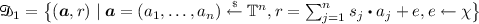.

### Definition 2 (GLWE problem over the torus)

Let $$N, k \in \mathbb {N}$$ with N a power of 2 and let. Let also $$\chi$$ be an error distribution over $$\mathbb {R}_N[X]$$. The general learning with errors (GLWE) over the torus problem is to distinguish the following distributions:

•;

•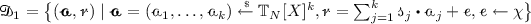.

The decisional LWE assumption (resp. the decisional GLWE assumption) asserts that solving the LWE problem (resp. GLWE problem) is infeasible for some security parameter $$\lambda$$, where $$n :=n(\lambda )$$ and $$\chi :=\chi (\lambda )$$ (resp. $$N :=N(\lambda )$$, $$k = k(\lambda )$$, and $$\chi :=\chi (\lambda )$$).

### B Algorithms

We use the notations of Sect. 4. The input of the (programmable) bootstrapping is anciphertext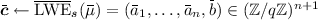that encrypts a plaintext $$\overline{\mu }\in \mathbb {Z}/q\mathbb {Z}$$ under the secret key $$\boldsymbol{s} = (s_1, \dots , s_n) \in \mathbb {B}^n$$.

### 1.1 B.1 Blind Rotation

The secret key bits $$s_j$$ used to encrypt the input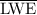ciphertext cannot be revealed. They are instead provided as bootstrapping keys; i.e., encrypted under some encryption key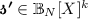: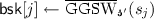for all $$j=1, \dots , n$$.

We then have:

At the end of the loop, $$\mathrm {ACC}$$ contains aencryption ofunder key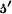.

### 1.2 B.2 Sample Extraction

The sample extraction algorithm extracts the constant coefficient $$\overline{\mu }$$ of polynomial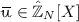in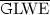ciphertext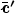as aciphertext of $$\overline{\mu }$$. In more detail, let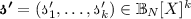with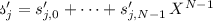for $$1 \le j\le k$$. Parsingaswith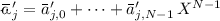for $$1\le j\le k$$ and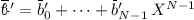, it can be verified that $$\boldsymbol{\overline{c}'} :=(\overline{a}'_{1,0}, -\overline{a}'_{1,N-1}, \dots , -\overline{a}'_{1,1}, \dots ,\overline{a}'_{k,0},-\overline{a}'_{k,N-1},\dots ,-\overline{a}'_{k,1}, \overline{b}'_0) \in (\mathbb {Z}/q\mathbb {Z})^{kN+1}$$ is a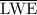encryption of $$\overline{\mu }$$ under the key $$\boldsymbol{s'} = (s'_1, \dots , s'_{kN}) \in \mathbb {B}^{kN}$$ where $$s'_{l+1+(j-1)N} :=s'_{j,l}$$ for $$1 \le j \le k$$ and $$0 \le l \le N-1$$.

### 1.3 B.3 Key Switching

The key switching technique can be used to switch encryption keys in different parameter sets [7, § 1.2]. Its implementation requires key-switching keys, i.e.,encryptions of the key bits of $$\boldsymbol{s}'$$ with respect to the original key $$\boldsymbol{s}$$. Assume we are given the key-switching keysfor all $$1 \le i \le kN$$ and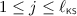, for some parametersanddefining a gadget decomposition (see Sect. 3.3). Adapting [10, § 4.1] teaches that, on inputciphertextunder the key $$\boldsymbol{s'} = (s_1, \dots , s_{kN}) \in \mathbb {B}^{kN}$$,

where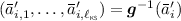is anencryption of $$\overline{\mu }$$ under key $$\boldsymbol{s}$$, provided that the resulting noise keeps small.

## Rights and permissions

Reprints and Permissions